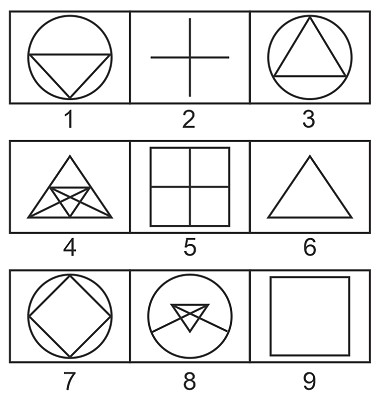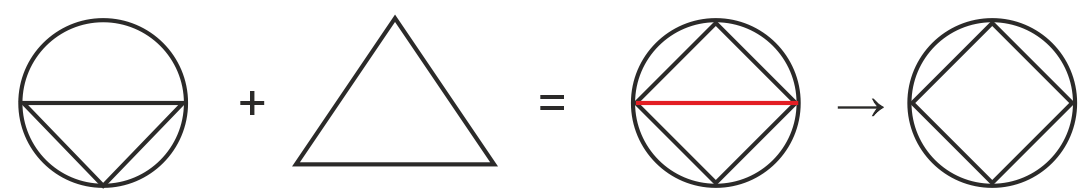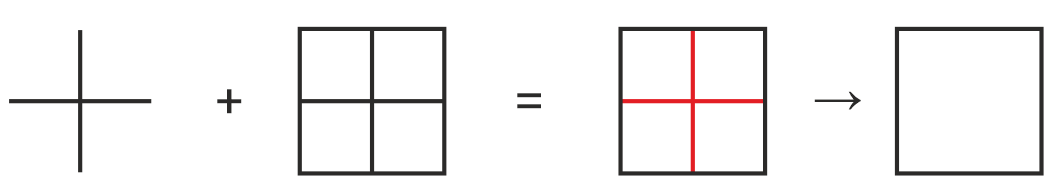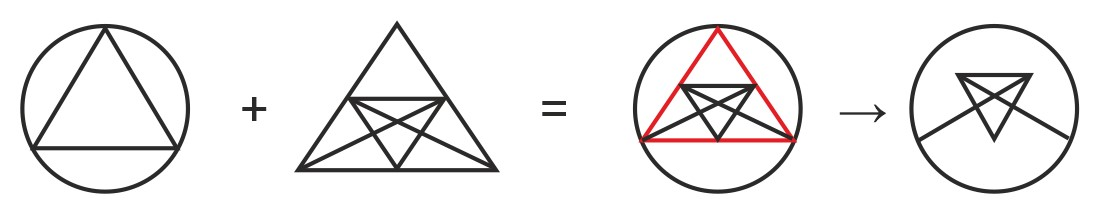# QOTD - Which of the following option will be correct if we group the given figures into three different classes such that no figure is taken more than once?

2019-08-10 | Team PendulumEduOptions

A. 1, 6, 9; 2, 5, 7 and 3, 4, 8

B. 1, 5, 7; 2,4, 8; and 3, 6, 9

C. 1, 6, 7; 2, 5, 9 and 3, 4, 8

D. 1, 4, 7; 2, 5, 8; and 3, 6, 9

Explanation:

Considering option C

1, 6, 7; 2, 5, 9 and 3, 4, 8

Combining figures 1 and 6, figure 7 can be obtained asSimilarly, figures 2 and 5 will result in figure 8And, figures 3 and 4 will result in figure 9Hence, C is the correct answer.

Such type of question is asked in the logical reasoning section of various competitive exams like CAT, MAT, XAT, RRB JE, RRB NTPC, etc. Try and attempt free mock tests of IBPS PO, IBPS SO, SSC CGL, SSC CHSL, SSC MTS and many more exams at PendulumEdu and learn new techniques to cope with typical logical reasoning questions. Also, clear your exams related doubts through PendulumEdu’s one-to-one session.0